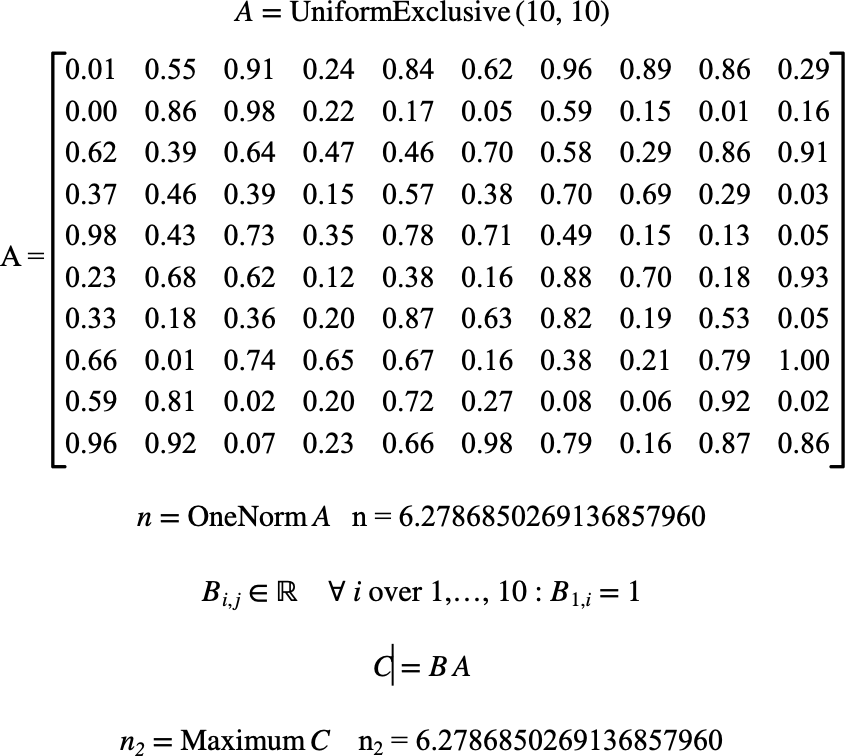# $$\text{OneNorm}$$¶

You can use the $$\text{OneNorm}$$ to calculate the one-norm of a matrix defined by:

You can use the \onorm backslash command to insert this function.

The following variants of this function are available:

• $$\text{real } \text{OneNorm} \left ( \text{<matrix>} \right )$$

Where $$\text{<matrix>}$$ is the matrix to calculate the one-norm of.

The function is implemented internally for real and complex matrices. Other matrix types will be converted to real matrices prior to calculating the norm.

The one-norm is calculated by:

$\text{OneNorm} \left ( M \right ) = \text{max} \left ( \sum_{r=1}^{ N _ r } \left \vert M _ { r, 1 } \right \vert , \sum_{r=1}^{ N _ r } \left \vert M _ { r, 2 } \right \vert , \ldots , \sum_{r=1}^{ N _ r } \left \vert M _ { r, N _ c } \right \vert \right )$

Where $$N _ r$$ is the number of rows in matrix $$M$$ and $$N _ c$$ is the number of columns in matrix $$M$$.

Figure 181 shows the basic use of the $$\text{OneNorm}$$ function.Figure 181 Example Use Of The OneNorm Function